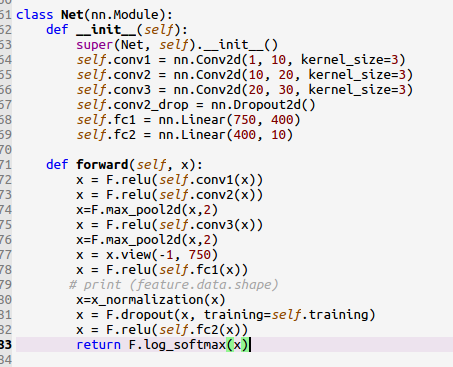# According to the each data of the batch ,normalize the output x

I want to normalize the output,for example,my batch size is 64 ,I will normalize for each line

``````def x_normalization(x):
num_pic,_=x.data.shape
for i in range(num_pic):
x[i,:]=x[i,:]/torch.max(x[i,:])    #normalize for each line
return x
``````

I call this function inI get a error
one of the variables needed for gradient computation has been modified by an inplace operation

Hi,

The problem is that you modify `x` in place while it’s value is needed to compute gradients.
You can replace your function with:

``````def x_normalization(x):
x = x / x.max(0, keepdim=True)
return x
``````

excuse me. I also encounter this problem when I run the code below in a forward() function(code is in the image). If I comment the `guess_img_dist_arr[n]=(torch.norm(guess_img[y][x]-guess_img[y_co][x_co]))`
It will not raise the error, but get a wrong result.
could you tell me how to fix this?

Hi,

You should not modify a single `Variable` inplace multiple times.
In your case, one wait to solve this would be:

``````for i in range(k_clusters):
# some code

guess_img_dist_list = []
for n in range(length):
# some code
guess_img_dist_list.append(torch.norm(...))

guess_img_dist_arr = torch.cat(guess_img_dist_list, 0)
``````

Thanks a lot!
You saved my day!

thanks, that is feasible